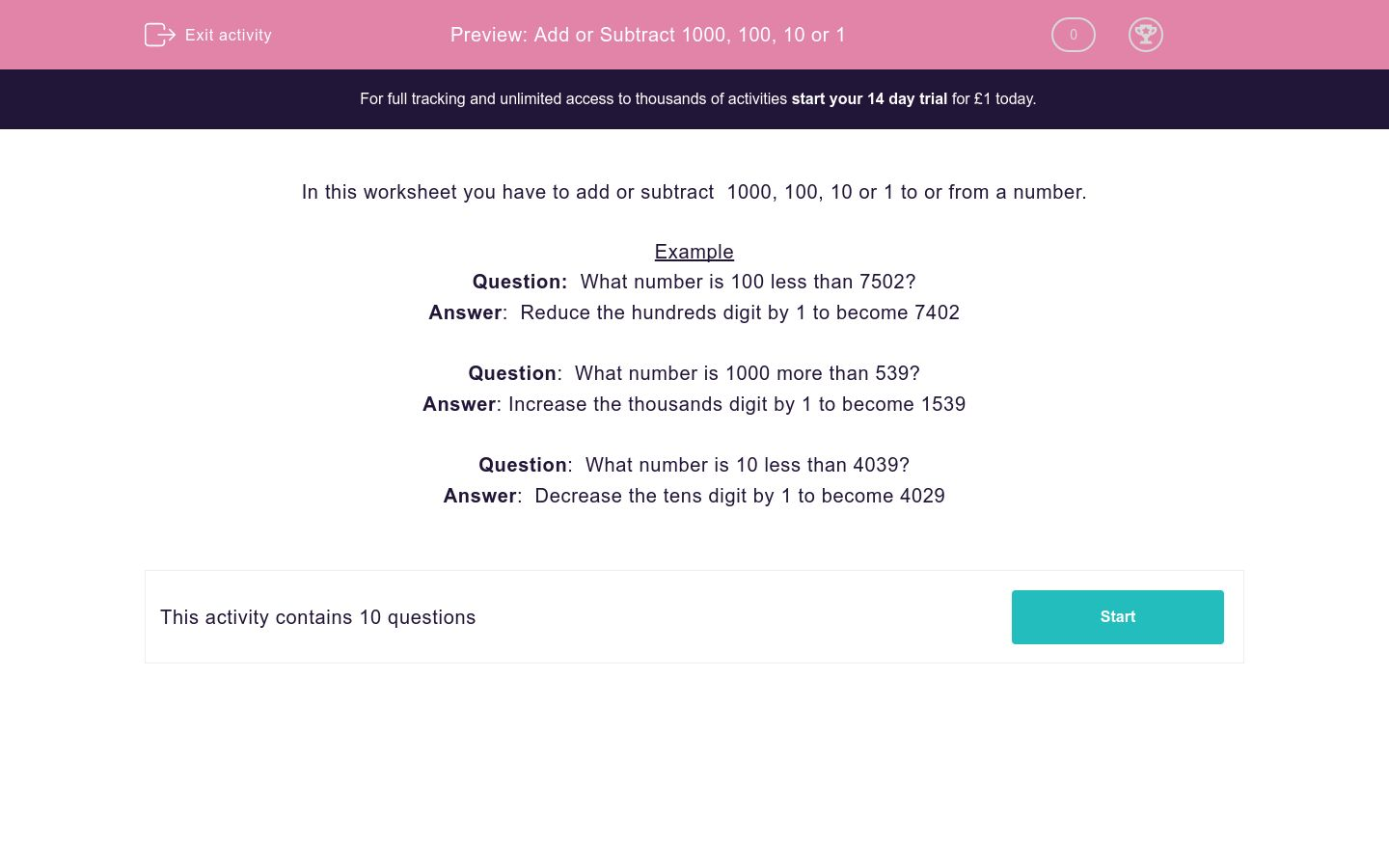# Add or Subtract 1000, 100, 10 or 1

In this worksheet, students add or subtract 1000, 100, 10 or 1 to/from a given number by looking at place value.Key stage:  KS 2

Curriculum topic:   Maths and Numerical Reasoning

Curriculum subtopic:   Mixed Problems

Difficulty level:#### Worksheet Overview

In this worksheet you have to add or subtract  1000, 100, 10 or 1 to or from a number.

Example

Question:  What number is 100 less than 7502?

Answer:  Reduce the hundreds digit by 1 to become 7402

Question:  What number is 1000 more than 539?

Answer: Increase the thousands digit by 1 to become 1539

Question:  What number is 10 less than 4039?

Answer:  Decrease the tens digit by 1 to become 4029

### What is EdPlace?

We're your National Curriculum aligned online education content provider helping each child succeed in English, maths and science from year 1 to GCSE. With an EdPlace account you’ll be able to track and measure progress, helping each child achieve their best. We build confidence and attainment by personalising each child’s learning at a level that suits them.

Get started